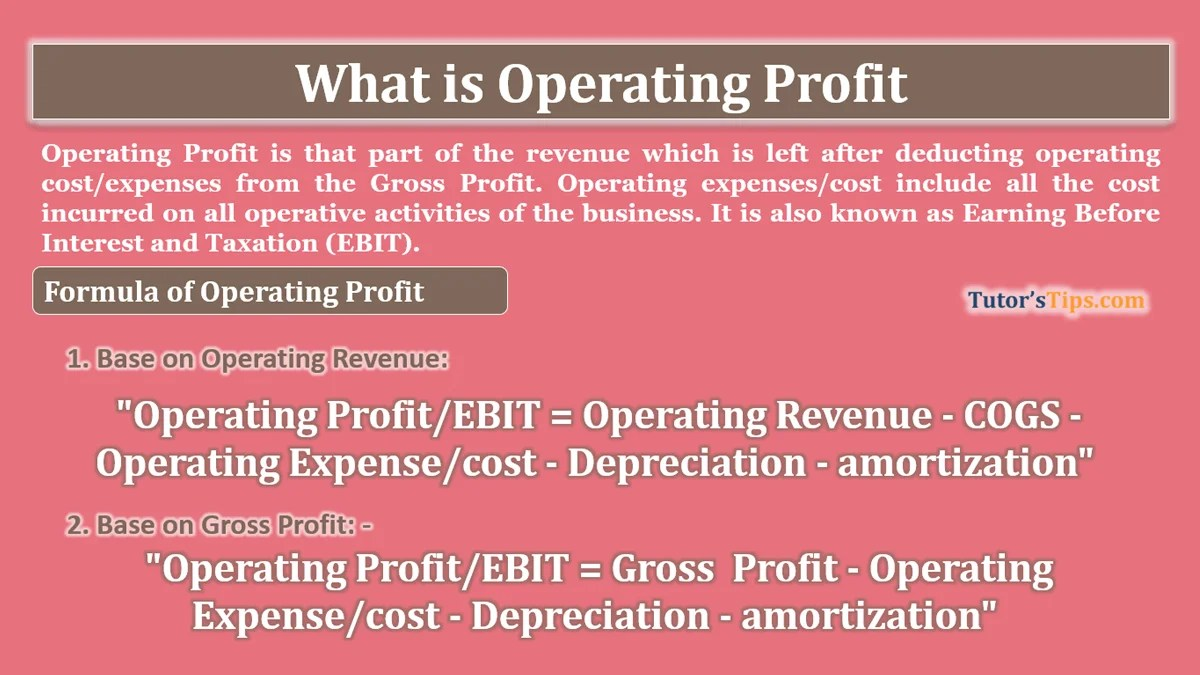# What is Operating profit – Explanation with ExamplesWhat-is-Operating Profit

Operating Profit is that part of the revenue which is left after deducting operating cost/expenses from the Gross Profit. Operating expenses/cost include all the cost incurred on all operative activities of the business. It is also known as Earning Before Interest and Taxation (EBIT).

### The Formula of Operating Profit : –

#### 1. Base on Operating Revenue:

EBIT can be calculated from the total operating revenue with the following formula:

Operating Profit/EBIT = Operating Revenue – COGS – Operating Expense/cost – Depreciation – amortization”

#### 2. Base on Gross Profit: –

EBIT can be calculated from the Gross profit with the following formula:

“Operating Profit/EBIT = Gross  Profit – Operating Expense/cost – Depreciation – amortization”

### Examples of Calculation of EBIT

#### Example 1 : –

As on 01/04/2019 A&b Co. ltd. have a stock of goods worth Rs. 35,000. The purchase goods worth Rs 1,50,000 and purchase return Rs 15,000 during the year and spent 2,000 on Carriage, 400 on loading. They sold goods for worth Rs. 5,35,000 and sale return Rs. 25,000 during the year and after that at the end of the year Rs 12,400 stock of goods left.

Other Expenses are salary 1,20,000/-, Electricity Rs. 50,000 and Depreciation of Plant and Machine Rs 10,000.

Calculate the EBIT of Mr X.

Solution: –

EBIT = Operating Revenue – COGS – Operating Expense/cost – Depreciation – amortization”

First, calculate Cost of Goods Sold: –

COGS =  Opening Stock + Net Purchase + Direct Expenses – Closing Stock

35000 + (150000 – 25000) + 2000 + 400 – 12400

COGS = 1,50,000/-

Operating Expenses = Salary  + Electricity
=1,20,000 + 50,000
Operating Expenses =1,70,000/-

EBIT    = (5,35,000 – 25,000) – 1,50,000 – 1,80,000
= 5,10,000 – 2,30,000
EBIT = 2,80,000/-

#### Example 2 : –

Gross Profit of the year is Rs 18,500 and  Further Mr X paid a salary to his employees 2,500/-, shop rent 1,000 and Shop lighting 200. Now calculate the EBIT earned by Mr X.

Solution: –

EBIT = Gross Profit + Operating Cost/ Expenses

We already got Gross Profit in the question = 18,500/-

SO,  18500 – 2500 -1000 – 200

EBIT = 14,800/-

#### Example 3 : –

Gross Profit of the year is Rs. 5,00,000, Further Mr Y paid a salary to his employees 92,500/-, Electricity charges Rs. 25,000 and Depreciation of Building Rs 50,000.  Now calculates the EBIT earned by Mr X.

Solution: –

EBIT = Gross Profit + Operating Cost/ Expenses

We already got Gross Profit in the question = 5,00,000/-

Operating Expenses =  Salary of Employee + Electricity Charges + Depreciation on Building
=  92,500 + 25,000 + 50,000
= 1,67,500/-

EBIT = 5,00,000 + 50,000 – 1,67,500

EBIT = 3,82,500/-

Check out the difference between Gross and Net Profit.

Difference between Gross profit and Net Profit

You can also read the following topics: –